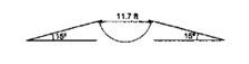Deepak Scored 45->99%ile with Bounce Back Crack Course. You can do it too!

# The given figure shows a 11.7 ft wide ditch with

Question:

The given figure shows a $11.7 \mathrm{ft}$ wide ditch with the approach roads at tan angle of $15^{\circ}$ with the horizontal. With what minimum speed should a motorbike be moving on the roads so that it safely crosses the ditch?

Assume that the length of the bike is $5 \mathrm{ft}$ and it leaves the road when the front part runs out of the approach road.Solution:

Range to be covered by bike $=11.7+5=16.7 \mathrm{ft}$

$\mathrm{R}=\frac{\frac{\mathrm{u}}{\sin 2 \theta}}{2 \mathrm{~g}}$

$16.6=\frac{u^{2} \sin (2 \times 15)}{2(32)}$

$\mathrm{u}=32 \mathrm{ft} / \mathrm{sec}$